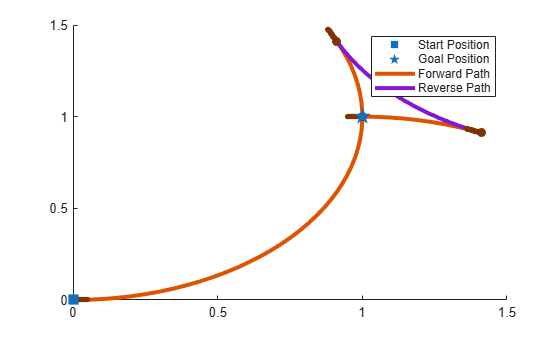# reedsSheppPathSegment

Reeds-Shepp path segment connecting two poses

## Description

The `reedSheppPathSegment` object holds information for a Reeds-Shepp path segment to connect between poses. A Reeds-Shepp path segment connects two poses as a sequence of five motion types. The motion options are:

• Straight

• Left turn at maximum steer

• Right turn at maximum steer

• No movement

## Creation

To generate a `reedSheppPathSegment` object, use the `connect` function with a `reedsSheppConnection` object:

`reedsPathSegObj = connect(connectionObj,start,goal)` connects the start and goal poses using the specified connection type object.

To specifically define a path segment:

`reedsPathSegObj = reedsSheppPathSegment(connectionObj,start,goal,motionLengths,motionTypes)` specifies the Reeds-Shepp connection type, the start and goal poses, and the corresponding motion lengths and types. These values are set to the corresponding properties in the object.

## Properties

expand all

Minimum turning radius of the vehicle, specified as a positive scalar in meters. This value corresponds to the radius of the turning circle at the maximum steering angle of the vehicle.

Data Types: `double`

Initial pose of the vehicle at the start of the path segment, specified as an [x, y, Θ] vector. x and y are in meters. Θ is in radians.

Data Types: `double`

Goal pose of the vehicle at the end of the path segment, specified as an [x, y, Θ] vector. x and y are in meters. Θ is in radians.

Data Types: `double`

Length of each motion in the path segment, specified as a five-element numeric vector in meters. Each motion length corresponds to a motion type specified in `MotionTypes`.

Data Types: `double`

Type of each motion in the path segment, specified as a five-element string cell array.

Motion TypeDescription
`"S"`Straight (forward, `p` or reverse, `n`)
`"L"`

Left turn at the maximum steering angle of the vehicle (forward, `p` or reverse, `n`)

`"R"`

Right turn at the maximum steering angle of the vehicle (forward, `p` or reverse, `n`)

`"N"`No motion

If a path segment has fewer than five motion types, the remaining elements are `"N"` (no motion).

Example: `{"L","S","R","L","R"}`

Data Types: `cell`

Direction of each motion in the path segment, specified as a five-element vector of 1s (forward motion) and –1s (reverse motion). Each motion direction corresponds to a motion length specified in `MotionLengths` and a motion type specified in `MotionTypes`.

When no motion occurs, that is, when a `MotionTypes` value is `"N"`, then the corresponding `MotionDirections` element is `1`.

Example: `[-1 1 -1 1 1]`

Data Types: `double`

Length of the path segment, specified as a positive scalar in meters. This length is just a sum of the elements in `MotionLengths`.

Data Types: `double`

## Object Functions

 `interpolate` Interpolate poses along path segment `show` Visualize path segment

## Examples

collapse all

Create a `reedsSheppConnection` object.

`reedsConnObj = reedsSheppConnection;`

Define start and goal poses as `[x y theta]` vectors.

```startPose = [0 0 0]; goalPose = [1 1 pi];```

Calculate a valid path segment to connect the poses.

`[pathSegObj,pathCosts] = connect(reedsConnObj,startPose,goalPose);`

Show the generated path.

`show(pathSegObj{1})`Reeds, J. A., and L. A. Shepp. "Optimal Paths for a Car That Goes Both Forwards and Backwards." Pacific Journal of Mathematics. Vol. 145, Number 2, 1990, pp. 367–393.